Fractal Basics

Learning Outcomes

• Define and identify self-similarity in geometric shapes, plants, and geological formations
• Generate a fractal shape given an initiator and a generator

Mathematical processes: The pencil-and-paper approach

Mathematical processes are often challenging to grasp. It can take several times reading through a text, watching a demonstration, and practicing the steps yourself before the idea becomes clear. But the time and effort spent in persisting through the challenge does eventually pay off.

When trying to obtain the understanding of an unfamiliar process, you may find it helpful to repeatedly write it out on paper.

This module contains several such processes and several different styles of demonstration of these processes: in words, images, worked-out solutions, and videos. Try employing the pencil-on-paper strategy as you work through each of the demonstrations and examples.

Remember to keep a list of new vocabulary along the way!

Fractals are mathematical sets, usually obtained through recursion, that exhibit interesting dimensional properties. We’ll explore what that sentence means through the rest of the chapter. For now, we can begin with the idea of self-similarity, a characteristic of most fractals.

Self-similarity

A shape is self-similar when it looks essentially the same from a distance as it does closer up.

Self-similarity can often be found in nature. In the Romanesco broccoli pictured below, if we zoom in on part of the image, the piece remaining looks similar to the whole.

Likewise, in the fern frond below, one piece of the frond looks similar to the whole.

Iterated Fractals

This self-similar behavior can be replicated through recursion: repeating a process over and over.

Example

Suppose that we start with a filled-in triangle. We connect the midpoints of each side and remove the middle triangle. We then repeat this process.If we repeat this process, the shape that emerges is called the Sierpinski gasket. Notice that it exhibits self-similarity—any piece of the gasket will look identical to the whole. In fact, we can say that the Sierpinski gasket contains three copies of itself, each half as tall and wide as the original. Of course, each of those copies also contains three copies of itself.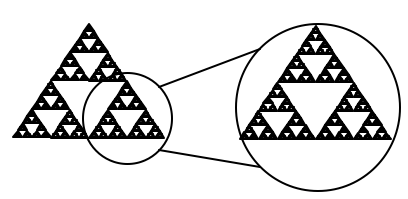In the following video, we present another explanation of how to generate a Sierpinski gasket using the idea of self-similarity.

We can construct other fractals using a similar approach. To formalize this a bit, we’re going to introduce the idea of initiators and generators.

Initiators and Generators

An initiator is a starting shape

A generator is an arranged collection of scaled copies of the initiator

To generate fractals from initiators and generators, we follow a simple rule:

Fractal Generation Rule

At each step, replace every copy of the initiator with a scaled copy of the generator, rotating as necessary

This process is easiest to understand through example.

Example

Use the initiator and generator shown to create the iterated fractal.This tells us to, at each step, replace each line segment with the spiked shape shown in the generator. Notice that the generator itself is made up of 4 copies of the initiator. In step 1, the single line segment in the initiator is replaced with the generator. For step 2, each of the four line segments of step 1 is replaced with a scaled copy of the generator:This process is repeated to form Step 3. Again, each line segment is replaced with a scaled copy of the generator.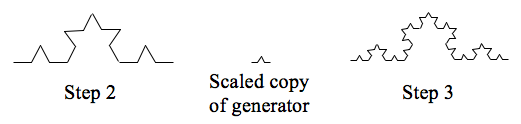Notice that since Step 0 only had 1 line segment, Step 1 only required one copy of Step 0.

Since Step 1 had 4 line segments, Step 2 required 4 copies of the generator.

Step 2 then had 16 line segments, so Step 3 required 16 copies of the generator.

Step 4, then, would require $16\cdot4=64$ copies of the generator.The shape resulting from iterating this process is called the Koch curve, named for Helge von Koch who first explored it in 1904.

Notice that the Sierpinski gasket can also be described using the initiator-generator approach.

Example

Use the initiator and generator below, however only iterate on the “branches.” Sketch several steps of the iteration.We begin by replacing the initiator with the generator. We then replace each “branch” of Step 1 with a scaled copy of the generator to create Step 2.Step 1, the generator. Step 2, one iteration of the generator.

We can repeat this process to create later steps. Repeating this process can create intricate tree shapes.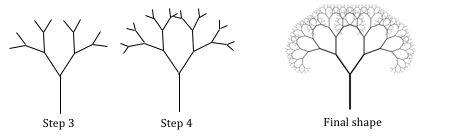Try It

Use the initiator and generator shown to produce the next two stages.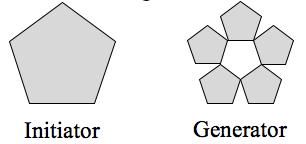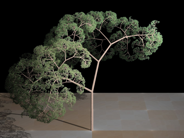Using iteration processes like those above can create a variety of beautiful images evocative of nature.

More natural shapes can be created by adding in randomness to the steps.

Example

Create a variation on the Sierpinski gasket by randomly skewing the corner points each time an iteration is made.

Suppose we start with the triangle below. We begin, as before, by removing the middle triangle. We then add in some randomness.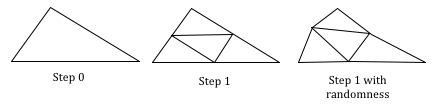We then repeat this process.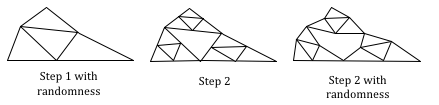Continuing this process can create mountain-like structures. This landscape was created using fractals, then colored and textured.The following video provides another view of branching fractals, and randomness.